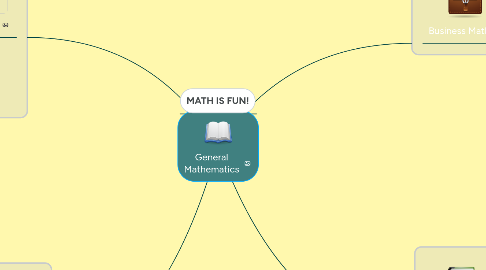# General Mathematics

Get Started. It's FreeGeneral Mathematics## 1. Functions

### 1.1. Linear Function

1.1.1. f(x) = 2x+3

1.2.1. f(x) = x^2 + 4x + 4

1.3.1. f(x) =2^x

### 1.4. Logarithmic Function

1.4.1. f(x) = ln(x+3)

1.5.1. P=2L + 2W

### 2.1. Simple and Compound Interest

2.1.1. I=PRT & [P (1 + i)n] – P

### 2.2. Ordinary, Defined, and General Annuity

2.2.1. P = PMT [(1 - (1 / (1 + r)n)) / r]

### 2.3. Ammortization

2.3.1. A = P (r(1+r)^n)/((1+r)^n -1)

## 3. Systems of Equation

### 3.1. Linear

3.1.1. y = 3x – 2 y = –x – 6

3.2.1. x^2 + y2 = 26    x - y = 6

## 4. Inequalities

4.1.1. 2x<9

### 4.2. Rational Inequalities

4.2.1. (x^2 + 3x + 2 )/(x^2 - 16) >=0

### 4.3. Logarithmic Inequalities

4.3.1. log3(3-2x)>=log3(4x+1)